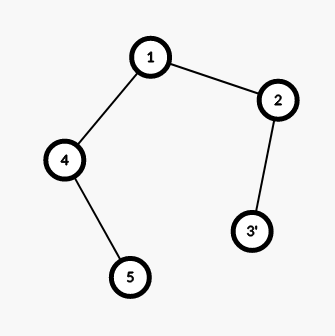Tree query
/

## Advanced Data Structures, Algorithms, Data Structures, Segment Trees, Trees

Problem
Editorial
Analytics

A tree is a simple graph in which every two vertices are connected by exactly one path. You are given a rooted tree with $n$ vertices and a lamp is placed on each vertex of the tree.

You are given $q$ queries of the following two types:

• $1\ v$: You switch the lamp placed on the vertex $v$, that is, either from On to Off or Off to On.
• $2\ v$: Determine the number of vertices connected to the subtree of $v$ if you only consider the lamps that are in On state. In other words, determine the number of vertices in the subtree of $v$, such as $u$, that can reach from $v$ by using only the vertices that have lamps in the On state.

Note: Initially, all the lamps are turned On and the tree is rooted from vertex number $1$.

Input format

• First line: Two integers $n$ and $q$ denoting the number of vertices and queries
• Next $n -1$ lines: Two number $a_i$ and $b_i$ denoting an edge between the vertices $a_i$ and $b_i$
• Next $q$ lines: Two integers $t_i$ and $v_i$, where $t_i$ is the type of the $i^{th}$ query

Note: It is guaranteed that these edges form a tree rooted from vertex $1$.

Output format
For every query of type 2, print the answer in a single line.

Constraints

$1 \leq n, q \leq 5 \times 10^{5}\\ 1 \leq a_i, b_i \leq n\\ 1 \leq t_i \leq 2\\ 1 \leq v_i \leq n$

SAMPLE INPUT
5 4
1 2
2 3
1 4
4 5
1 3
2 2
1 3
2 2

SAMPLE OUTPUT
1
2

ExplanationThe tree after turning off lamp in node $3$

Obviously the answer now for vertex $2$ is $1$

Time Limit: 4.0 sec(s) for each input file.
Memory Limit: 256 MB
Source Limit: 1024 KB

## This Problem was Asked in

Initializing Code Editor...